Open in App
Not now

# Class 10 NCERT Solutions- Chapter 8 Introduction To Trigonometry – Exercise 8.1

• Last Updated : 11 Dec, 2020

### (i) sin A, cos A (ii) sin C, cos C

Solution: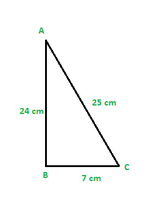Using Pythagoras theorem for ΔABC

AC2 = AB2 + BC2

= (24 cm)2 + (7 cm)2

= (576 + 49) cm2

= 625 cm2

∴AC = 25 cm

(i) sin A = opp/hyp

sin A = 7/25

cos A = adj/hyp = 24/25

cos A = 24/25

(ii) sin C = opp/hyp

sin C = 24/25

cos C = 7/25

### Question 2. In Fig. 8.13, find tan P – cot R.

Solution: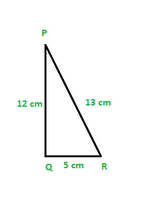Applying Pythagoras theorem for ΔPQR, we obtain

PR2 = PQ2 + QR2

(13 cm)2 = (12 cm)2 + QR2

169 cm2 = 144 cm2 + QR2

25 cm2 = QR2

QR = 5 cm

tan P = 5/12

cot R = 5/12

tan P – cot R = 5/12 – 5/12 = 0

### Question 3. If sin A = 3/4, calculate cos A and tan A.

Solution:

Using sin2A + cos2A = 1

(3/4)2 + cos2A = 1

cos2A = 1 – (3/4)2 = 1 – 9/16

cos A = 71/2/4

tan A = sin A/cos A

tan A = (3/4)/(71/2/4)

tan A = 3/71/2

### Question 4: Given 15 cot A = 8. Find sin A and sec A

Solution:

Given, 15 cot A = 8

cot A = 8/15

tan A = 1/cot A

tan A = 15/8

Using, 1 + tan2A = sec2A

1 + (15/8)2 = sec2A

289/64 = sec2A

sec A = 17/8

We know, cos2A = 1/sec2A

cos2A = 64/289

sin2A = 1 – cos2A

sin2A = 225/289

sin A = 15/17

### Question 5: Given sec θ = 13/12, calculate all other trigonometric ratios.

Solution.

Using Pythagoras theorem,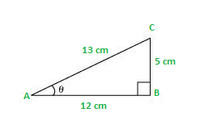sin θ = 5/13

cos θ = 12/13

tan θ = 5/12

cosec θ = 13/5

cot θ = 12/5

### Question 6: If ∠A and ∠B are acute angles such that cos A = cos B, then show that ∠A = ∠B.

Solution:

Let us consider a ΔABC in which CD ⊥ AB.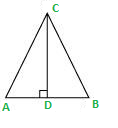It is given that cos A = cos B

We need to prove ∠A = ∠B. To prove this, we need to extend AC to P such that BC = CP.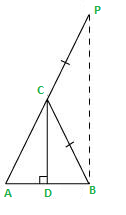From equation (1), we obtain

AD/BD = AC/CP    (BC = CP by construction)

By using the converse of B.P.T (Basic Proportionality Theorem),

CD||BP

∠ACD = ∠CPB (Corresponding angles) … (3)

And, ∠BCD = ∠CBP (Alternate interior angles) … (4)

By construction, we have BC = CP.

∴ ∠CBP = ∠CPB (Angle opposite to equal sides of a triangle) … (5)

From equations (3), (4), and (5), we obtain

∠ACD = ∠BCD … (6)

∠ACD = ∠BCD (Using equation (6))

∠CDA = ∠CDB (Both 90°)

Therefore, the remaining angles should be equal.

⇒ ∠A = ∠B

### (ii) cot2θ

Solution:

(i) Using (a + b) * (a – b) = a2 – b2 in numerator and denominator

We get

(1 – sin2θ)/(1 – cos2θ)

Using sin2θ + cos2θ = 1

We get

cos2θ/sin2θ = cot2θ

Now

cot2θ = (7/8)2 = 49/64

(ii) cot2θ = (7/8)2 = 49/64

### Question 8. If 3 cot A = 4, Check whether (1 – tan2A)/(1 + tan2A) = cos2A – sin2A

Solution.

We know that, tanA = sinA / cosA   ….(1)

Using (1) on L.H.S

= (1 – sin2A/cos2A)/(1 + sin2A/cos2A)

which on rearranging becomes

= (cos2A – sin2A)/(cos2A + sin2A)

Using the identity,

cos2A + sin2A = 1

LHS becomes

= (cos2A – sin2A)

This is equal to RHS.

LHS = RHS (for every value of cot A)

Hence, Proved.

### (ii) cos A cos C − sin A sin C

Solution:

Using Pythagoras theorem

(AB)2 + (BC)2 = (AC)2

(31/2)2 + (1)2 = (AC)2

which gives

AC = 2 cm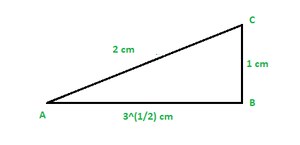Using formulas

sin A = 1/2

sin C = 31/2/2

cos A = 31/2/2

cos C = 1/2

Now, (i) sin A cos C + cos A sin C

Substituting the values

= (1/2) * (1/2) + (31/2/2) * (31/2/2)

= 1/4 + 3/4

= 1

Now, (ii) cos A cos C − sin A sin C

Substituting the values

= (31/2/2) * (1/2) – (1/2) * (31/2/2)

= 31/2/4 – 31/2/4

= 0

### Question 10: In ΔPQR, right-angled at Q, PR + QR = 25 cm and PQ = 5 cm. Determine the values of sin P, cos P and tan P.

Solution:

Given that, PR + QR = 25

PQ = 5

Let PR be x cm.

Therefore, QR = 25 − x cm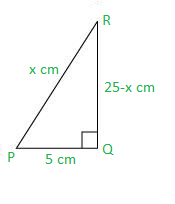Applying Pythagoras theorem in ΔPQR, we obtain

PR2 = PQ2 + QR2

x2 = (5)2 + (25 − x)2

x2 = 25 + 625 + x2 − 50x

50x = 650

x = 13

Therefore, PR = 13 cm

QR = (25 − 13) cm = 12 cm

Now,

sin P = QR/PR = 12/13

cos P = PQ/PR = 5/13

tan P = QR/PQ = 12/5

### (v) sin θ = 4/3, for some angle θ

Solution:

(i) Consider a ΔPQR, right-angled at Q as shown below.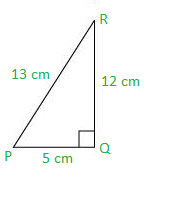Here tan P = 12/5 which is surely greater than 1.

Therefore, the statement is false.

(ii) Consider ΔABC with AB = 5 cm, AC = 12 cm and BC = x cm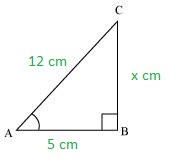Using Pythagoras theorem in ΔABC

(AB)2 + (BC)2 = (AC)2

52 + x2 = 122

x = (144 – 25)1/2

x = (119)1/2

x = 10.9 cm

AB < BC < AC

So this triangle is valid,

Therefore, given statement is true.

(iii) Abbreviation used for cosecant A is cosec A. And cos A is the abbreviation used for cosine A.

Hence, the given statement is false.

(iv) cot A is not the product of cot and A. It is the cotangent of ∠A.

Hence, the given statement is false.

(v) sin θ = 4/3

In a right-angled triangle, hypotenuse is always greater than the remaining two sides. Therefore, such value of sin θ is not possible.

Hence, the given statement is false

My Personal Notes arrow_drop_up
Related Articles